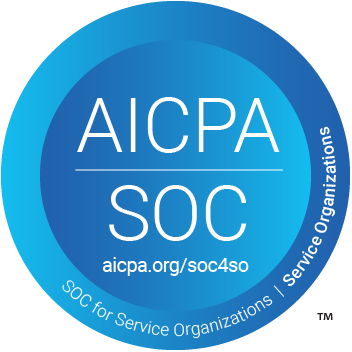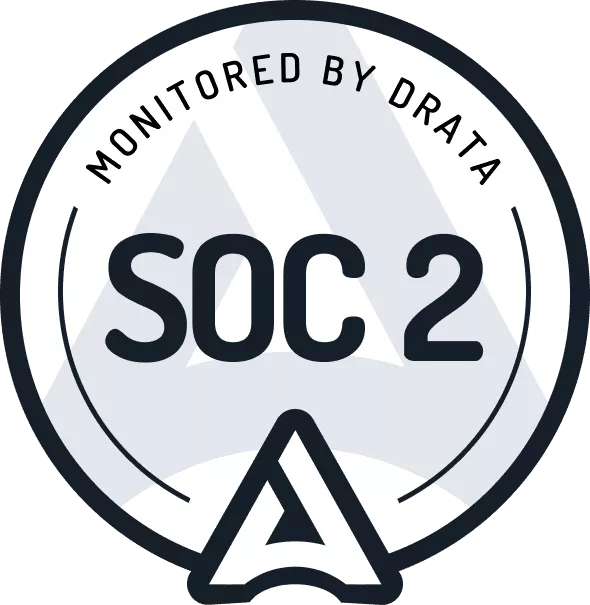# How to Calculate a Lease Liability using Excel

The lease liability is the present value of the known future lease payments at a point in time. A lease liability is required to be calculated for both ASC 842 & IFRS 16.

Refer below for seven steps on how to calculate the lease liability using excel’s goal seek. The lease liability we’re going to calculate is based on the following terms:

• 12-year lease term
• \$10,000 payments at the beginning of each year
• Discount rate: 7%

## Step 1 - Create a spreadsheet and set up columns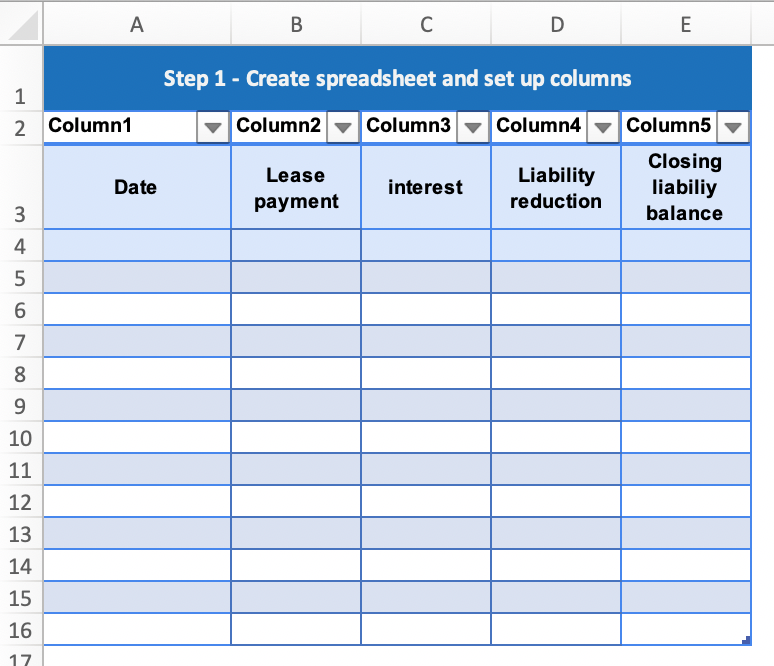In the new excel spreadsheet and name the five columns:

• Date
• Lease payment
• Interest
• Liability reduction
• Closing liability balance

## Step 2 - Enter the payment amounts and the payment dates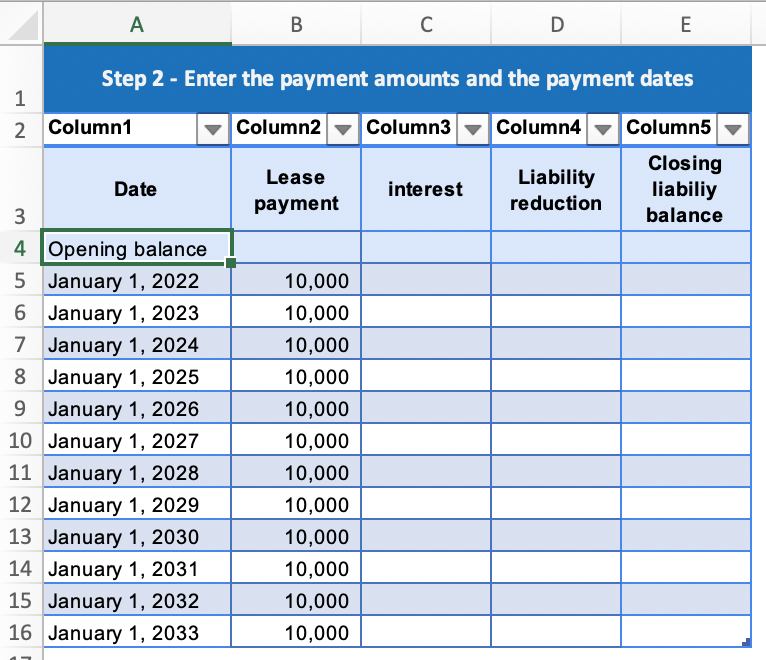Each row will include the date of the payment and the payment amount for the life of the lease.

## Step 3 - Calculate the interest on each payment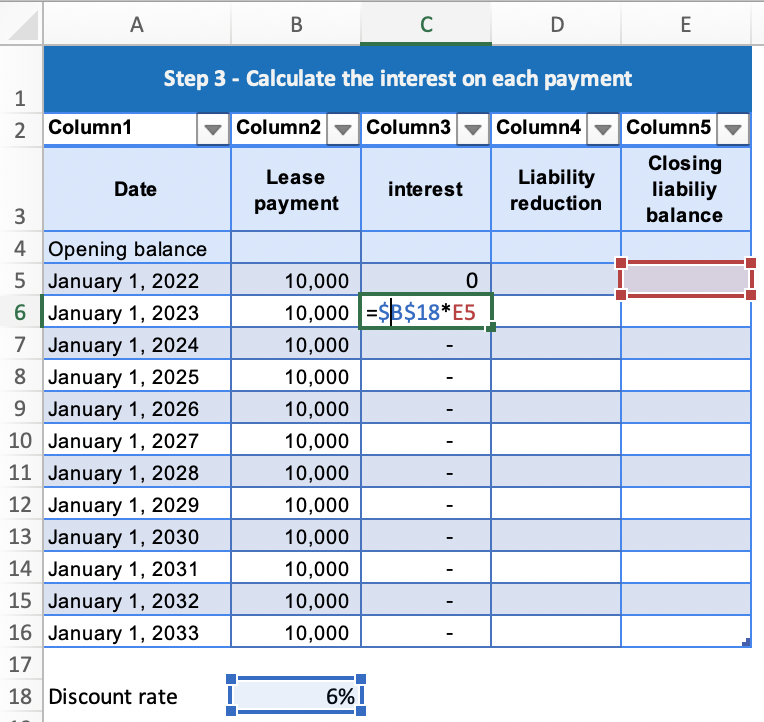Calculate the interest incurred on each payment using the discount rate of 6%. For the first payment, there is no interest incurred as it’s made at the commencement of the lease.

## Step 4 - Calculate the reduction of the lease liability for each payment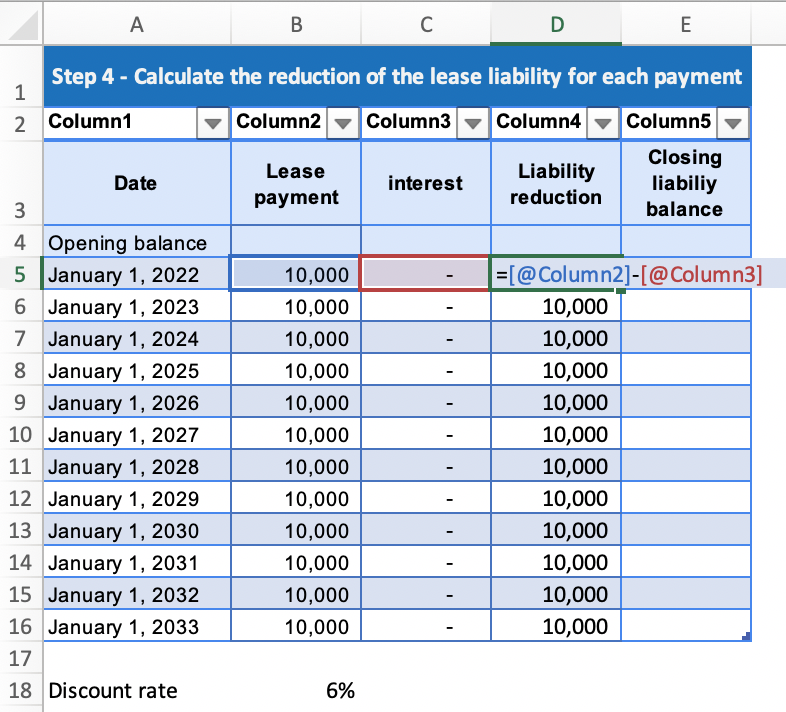The lease payment amount, less the interest occurred is the amount that the lease liability balance will be reduced.

## Step 5 - Input the formula to calculate the closing balance of the lease liability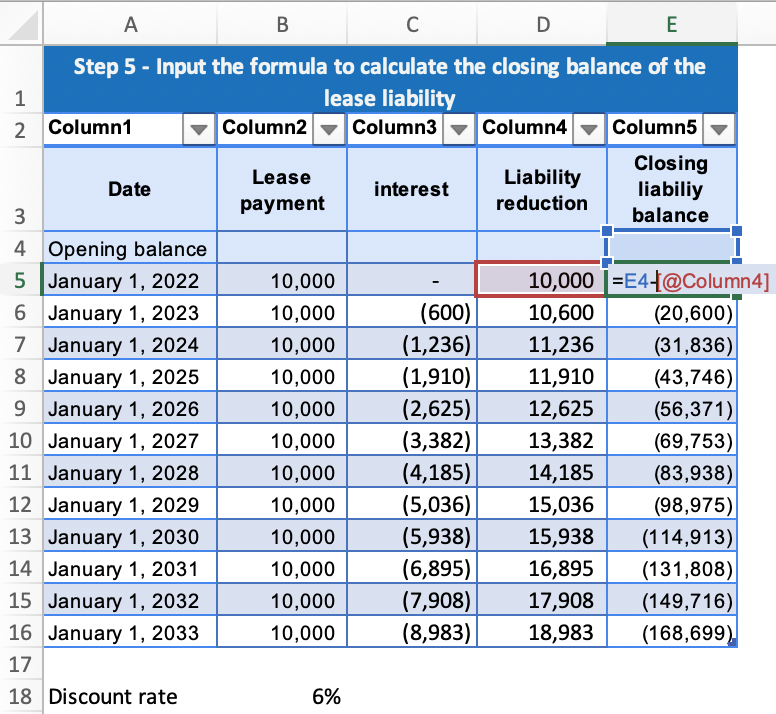This formula is the previous balance less the liability reduction amount to give the closing balance of the lease liability after the payment.

## Step 6 - Calculate the opening balance of the lease liability using excel’s goal seek function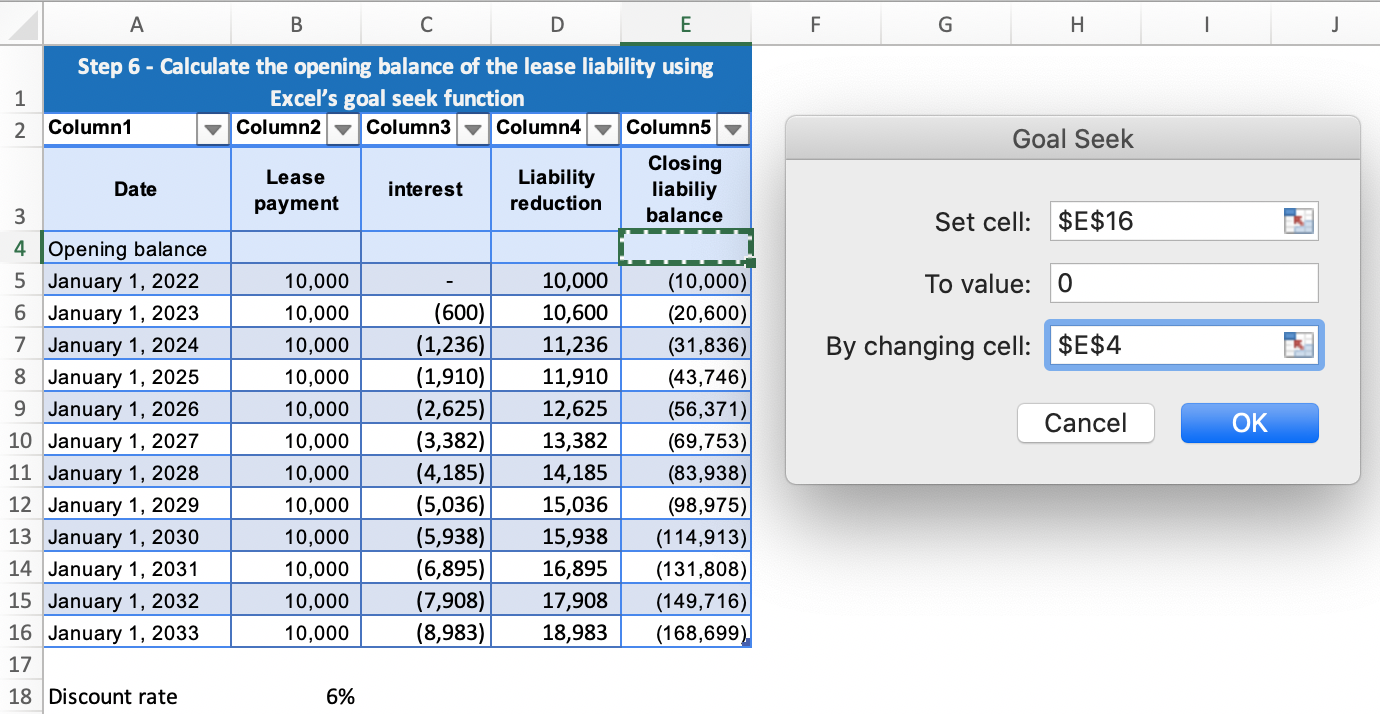This will calculate the opening balance amount of the lease liability. From this amount, the lease liability will unwind to zero. To do this in excel, select Data > What-if Analysis > Goal Seek.

## Step 7 - You’re done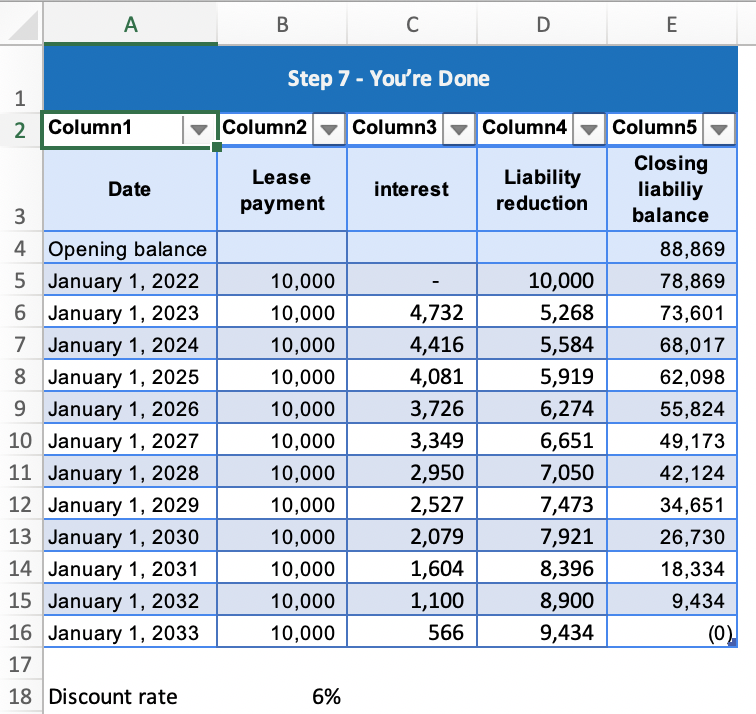Ensure the balance unwinds to zero. If it does, you have calculated the lease liability using goal seek.

## Conclusion

This is one method of calculating the lease liability. I would highly recommend reading How to Calculate the Present Value of Future Lease Payments to understand there are more and arguably more accurate methods when calculating the lease liability.

## FooterHere at Cradle, our mission is simple; it's at the foundation of everything that we do. We want to make accountants' lives easier by leveraging technology to free up their time to focus on running the business.

140 Yonge St.
Suite 200
Toronto, ON M5C 1X6

US
Suite #73591
Torrance, CA 90503
USA

### Legal